Nagoya Mathematical Journal ( IF 0.556 ) Pub Date : 2018-03-06 , DOI: 10.1017/nmj.2018.11
HONG RAE CHO; SOOHYUN PARK

Let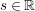$s\in \mathbb{R}$ and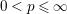$0<p\leqslant \infty$ . The fractional Fock–Sobolev spaces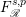$F_{\mathscr{R}}^{s,p}$ are introduced through the fractional radial derivatives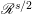$\mathscr{R}^{s/2}$ . We describe explicitly the reproducing kernels for the fractional Fock–Sobolev spaces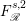$F_{\mathscr{R}}^{s,2}$ and then get the pointwise size estimate of the reproducing kernels. By using the estimate, we prove that the fractional Fock–Sobolev spaces$F_{\mathscr{R}}^{s,p}$ are identified with the weighted Fock spaces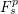$F_{s}^{p}$ that do not involve derivatives. So, the study on the Fock–Sobolev spaces is reduced to that on the weighted Fock spaces.

down
wechat
bug